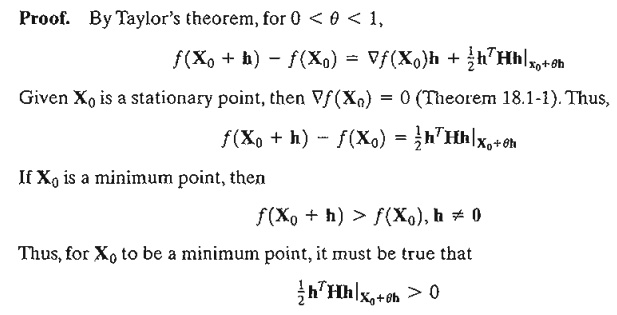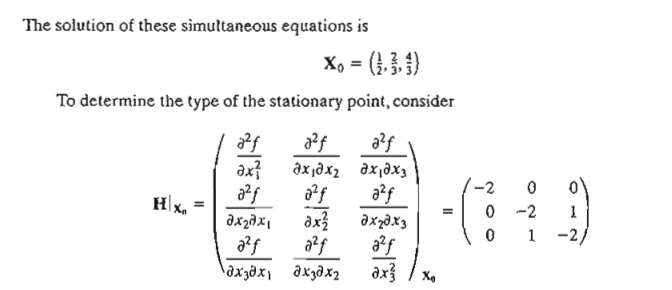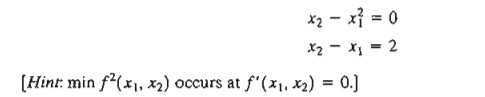Home | | Resource Management Techniques | Necessary and Sufficient Conditions -Unconstrained Problems

# Necessary and Sufficient Conditions -Unconstrained Problems

This section develops the necessary and sufficient conditions for an n-variable function f(X) to have extrema.

Necessary and Sufficient Conditions

This section develops the necessary and sufficient conditions for an n-variable function f(X) to have extrema. It is assumed that the first and second partial derivatives of f(X) are continuous for all X.We show by contradiction that Ñf(X o) must vanish at a minimum point Xo. For suppose it does not, then for a specific j the following condition will hold.This result contradicts the assumption that X o is a minimum point. Thus, Ñf(X o) must equal zero. A similar proof can be established for the maximization case.

Because the necessary condition is also satisfied for inflection and saddle points, it is more appropriate to refer to the points obtained from the solution of Ñf(Xo) = 0 as stationary points. The next theorem establishes the sufficiency conditions for X o to be an extreme point.

Theorem 18.1-2. A sufficient condition for a stationary point X o to be an extremum is that the Hessian matrix H evaluated at X o satisfy the following conditions:

i.            is positive definite if Xois a minimum point.

ii.            is negative definite I fXo is a maximum point.

Proof. By Taylor's theorem, for 0 q < 1,Given that the second partial derivative is continuous, the expression  ½  hTHh must have the same sign at both Xo and Xo + qh. Because hTHhl Xo defines a quadratic form (see Section 0.3 on the CD), this expression (and hence hTXh|Xo+qh) is positive if and only if, H|x0 is positive-definite. This means that a sufficient condition for the stationary point X o to be a minimum is that the Hessian matrix, H, evaluated at the same point is positive-definite. A similar proof for the maximization case shows that the corresponding Hessian matrix must be negative-definite.The principal minor determinants of H|xo have the values -2,4, and -6, respectively. Thus, as shown in Section D.3, HIXu is negative-definite and Xo = (1/2, 2/3, 4/3) represents a maximum point.

In general, if H|x0 is indefinite, Xo must be a saddle point. For nonconclusive cases, Xo mayor may not be an extremum and the sufficiency condition becomes rather involved, because higher-order terms in Taylor's expansion must be considered.

The sufficiency condition established by Theorem 18.1-2 applies to single-variable functions as follows. Given that yo is a stationary point, then

i.            yo is a maximum if f” (yo)  < 0.

ii.            yo is a minimum if f" (yo)  > 0.

If f” (y0) = 0, higher-order derivatives must be investigated as the following theorem requires.Theorem 18.1-3. Given yo, a stationary point of f(y), if the first (n  - 1) derivatives are zero and f(n)(yo) != 0, then

i.            If n is odd, yo is an inflection point.

ii.            If n is even then yo is a minimum if f(n)(yo)  > 0 and a maximum if f(n)(yo)  < 0.

Example 18.1-2

Figure 18.2 graphs the following two functions:3. Verify that the functionhas the stationary points (0, 3, 1), (0, 1, -1), (1,2,0), (2, 1, 1), and (2, 3, -1). Use the sufficiency condition to identify the extreme points.

*4. Solve the following simultaneous equations by converting the system to a nonlinear objective function with no constraints.5. Prove Theorem 18.1-3.

Study Material, Lecturing Notes, Assignment, Reference, Wiki description explanation, brief detail
Operations Research: An Introduction : Classical Optimization Theory : Necessary and Sufficient Conditions -Unconstrained Problems |